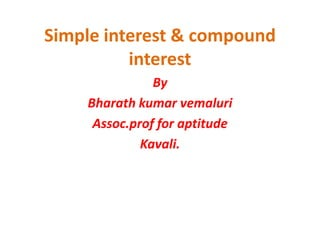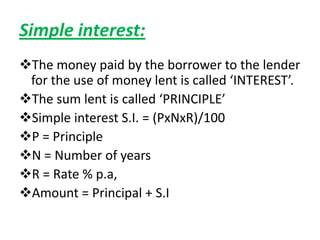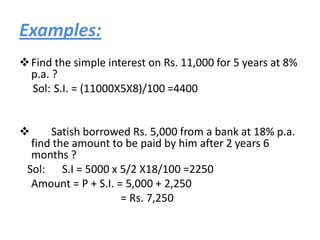Diese Präsentation wurde erfolgreich gemeldet.
Die SlideShare-Präsentation wird heruntergeladen. ×

# Simple interest & compound interest vedioWird geladen in …3
×

1 von 10
1 von 10

# Simple interest & compound interest vedio

simple interest and compound interest video is used to solve the sums in easy and quickly.

simple interest and compound interest video is used to solve the sums in easy and quickly.

Anzeige
Anzeige

## Weitere Verwandte Inhalte

Anzeige

### Simple interest & compound interest vedio

1. 1. Simple interest & compound interest By Bharath kumar vemaluri Assoc.prof for aptitude Kavali.
2. 2. Simple interest: The money paid by the borrower to the lender for the use of money lent is called ‘INTEREST’. The sum lent is called ‘PRINCIPLE’ Simple interest S.I. = (PxNxR)/100 P = Principle N = Number of years R = Rate % p.a, Amount = Principal + S.I
3. 3. Examples:  Find the simple interest on Rs. 11,000 for 5 years at 8% p.a. ? Sol: S.I. = (11000X5X8)/100 =4400  Satish borrowed Rs. 5,000 from a bank at 18% p.a. find the amount to be paid by him after 2 years 6 months ? Sol: S.I = 5000 x 5/2 X18/100 =2250 Amount = P + S.I. = 5,000 + 2,250 = Rs. 7,250
4. 4. Mr. Govind lent Rs. 1800 to Mr. Khan for 3 years and Rs. 3300 to Mr. Mukund for 2 years and he received Rs. 480 as simple interest in all. What is the rate of interest per annum? Sol: S.I. obtained by Mr. Govind = (1800X3XR)/100 + (3300X2XR)/100 = 54 R + 66 R = 120 R = Rs. 480 R = 4% p.a. A certain sum of money is invested by Mr. Gopal at 7% p.a. after 4 years. However he would get Rs. 520 more simple interest, if he invest the same sum a t6% p.a. for 9 years. What is the sum? Sol: The difference between S.I. obtained in two cases is Rs.520 ( PX9X6)/100 – (PX4X7)/100= 520 54P-28P=52000 26P=52000 p=52000/26 = 2000
5. 5.  In how many years will a sum of money become five times at 20% p.a. simple interest? Sol: Here Amount = 5P…………….(A=P+4P=5P) S.I. = 4P 4P = (PXNX20)/100 => N = 20 years
6. 6. Note:  in S.I the interest remains constant or is same for every year.  in S.I the interest percentages over the year can be added.  in C.I the interest percentages over the years are to be multiplied individually.
7. 7. Compound interest:  Calculate the C.I 0n 10000 at 20% per annum after 2 years. The interest being compounded annually? soln: p=10000 T=2 years R%=20% amount=10000X1.2X1.2=12400 C.I=12400-10000 = 2400
8. 8. Calculate the C.I on 10000 at 10% per annum after 1.5 years the interest being compounded halfyearly? Soln: p= 10000 T=1.5 years (6 months+ 6 months+ 6 months) R%=10% ,so for six months 5% Amount= 10000X1.05x1.05X1.05=11576.25 C.I= amount-principle=11576.25-10000=1576.25.  Arun deposited 8000 in HDFC bank which pays him 12% per annum for 12 months . the interest being compounded quarterly . find the amount? Soln: principle=8000 Time =1 year (3m+3m+3m+3m) quarter= 3 months. rate= 12% ,so 3% for every quarter amount= 8000X1.03X1.03X1.03X1.03 =9004.07
9. 9. The difference between C.I & S.I on a certain sum of money after 3 years,20% per annum is 2560. find the sum? soln: p= y T= 3 years R=20% Difference between c.i and s.i is 2560, 1.2X1.2X1.2X Y – 1.6 y=2560 1.728y-1.6y=2560 0.128y= 2560 y=20000/-(sum)
10. 10.  A sum of money becomes 4 times in 10 years . in how many years will it become 64 times on C.I? soln: *4 *4 *4 X…………………..4X……………………16X…………………..64X 10 yrs 10 yrs 10 yrs so 30 years.